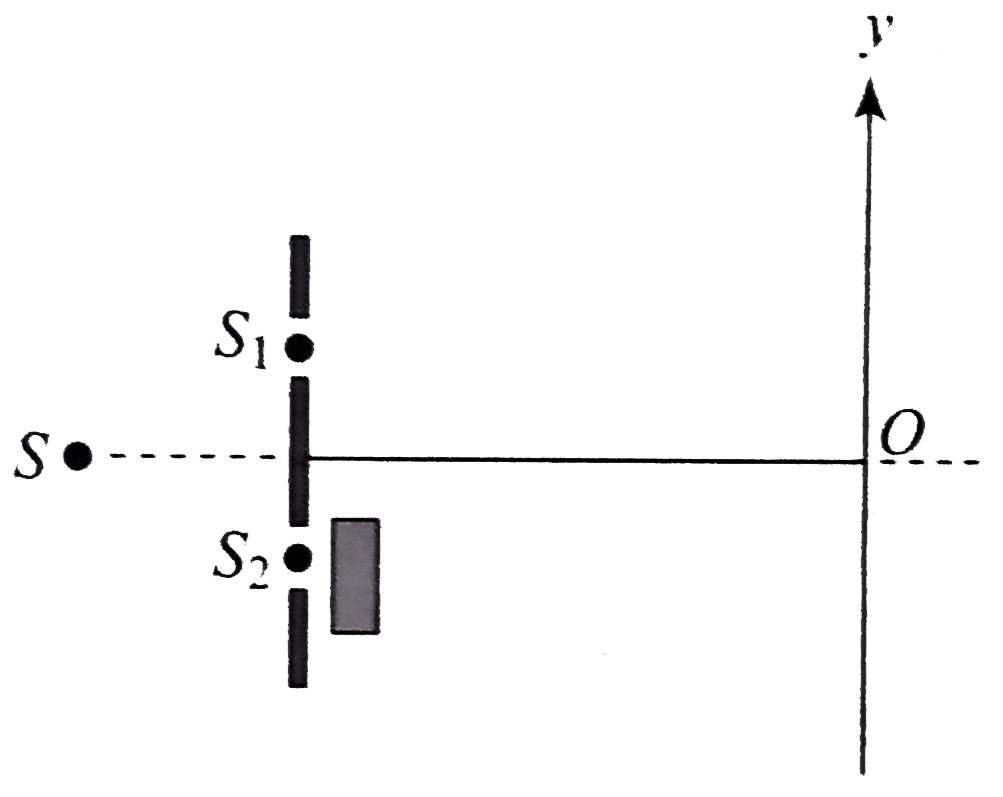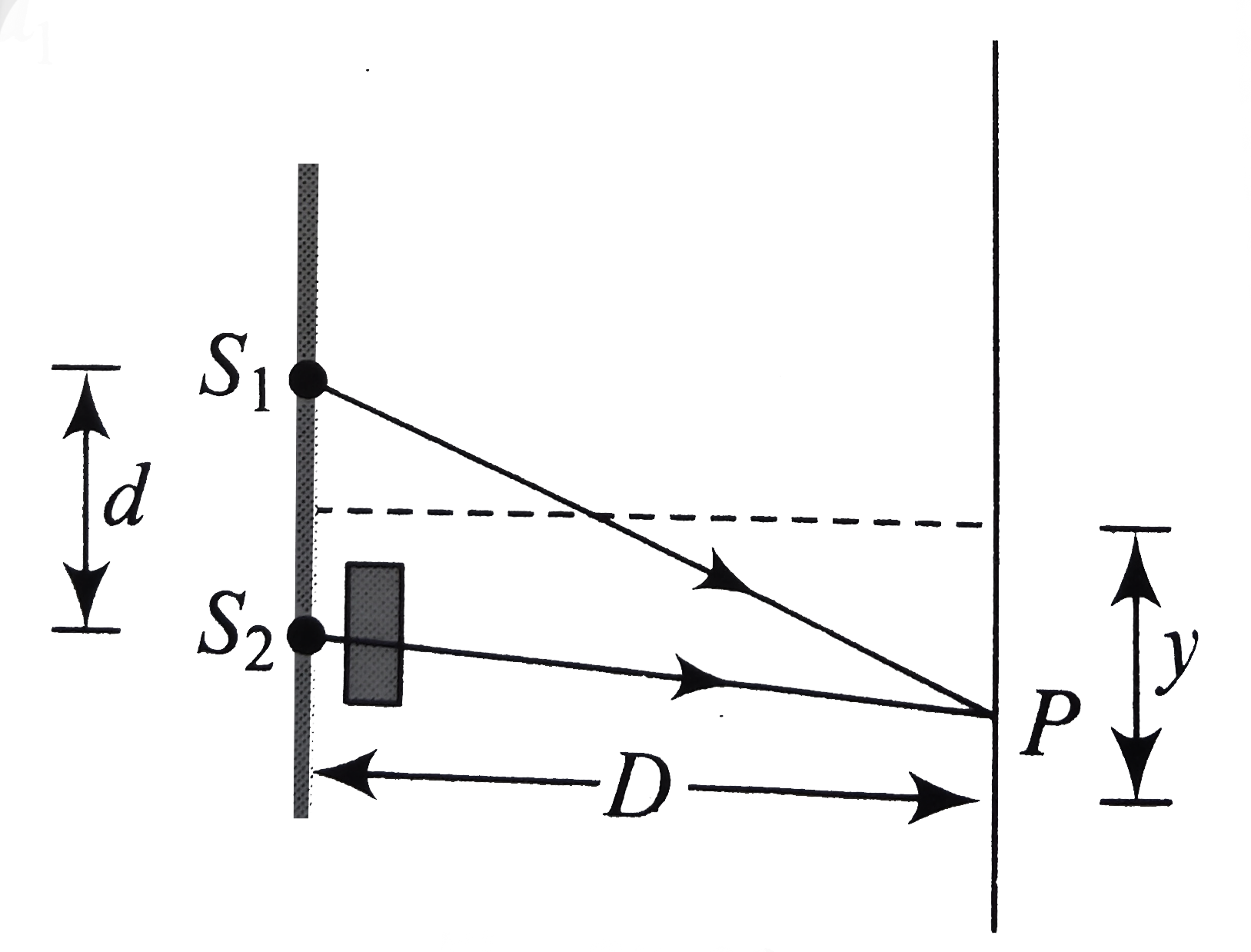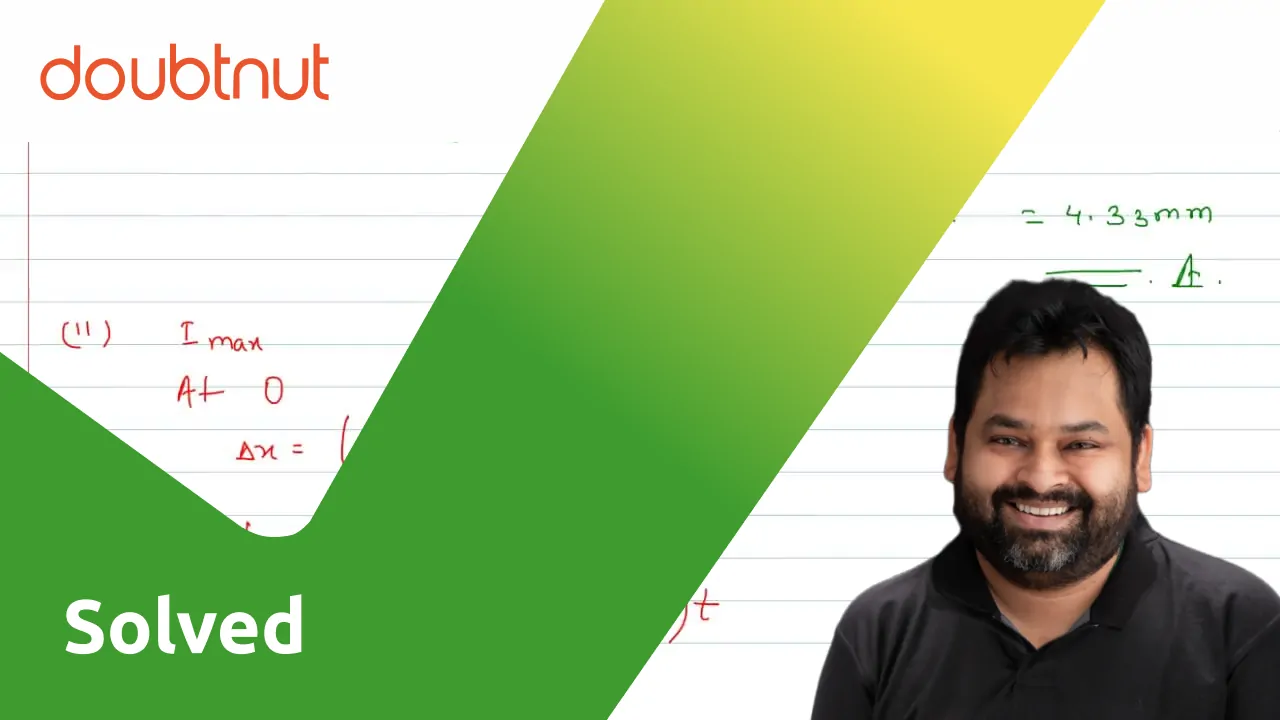Doubtnut App - Get 50 Lakh+ video solutions better than GoogleInstall Now

# A YDSE is performed in a medium of refractive index 4/3, A light of 600 nm wavelength is falling on the slits having 0.45 nm separation . The lower slit S2 is covered b a thin glass plate of thickness 10.4 mm and refractive index 1.5. The interference pattern is observed on a screen placed 1.5 m from the slits as shown in figure. (All the wavelengths in this problem are for the given medium of refractive index 4/3, ignore absorption.)Find the light intensity at point O relative t maximum fringe intensity.

A

14Imax

B

54Imax

C

12Imax

D

34Imax

Text Solution
Verified by Experts

## Path difference at point P on the screen, Δx=ydD At the position of central maxima, the optical path length S2P and S1P and equal. (S2P−t)c/μ1=1c/μ2=S1Pc/μ1 where μ1=4/3,μ2=3/2. μ1(S1P−S2P)=(μ2−μ)t μ1(ydD)=(μ2−μ1)t y=(μ2−μ1)tDμ1d =[(3/2)−(4/3)]×10.4×1.5(4.3)×0.45×10−3=4.33mmb. At point O, net path difference. Δx=(μ2μ1−1)t Net phase difference, Δϕ=2πλΔx =2π6×10−7(1.54/3−1)(10.4×10−6)=(133)π Thus, intensity I=Imaxcos2(ϕ/2) =Imaxcos2(13π6)=34Imax c. For maximum intensity at point O, Δx=nλ Path difference at point O, Δx=(1.54/3−1)(10.4×10−6)=1300nm Thus, maximum intensity will correspond to 13002nm, 13003nm,... In the given range, required values are 650 nm and 433.33 nm.